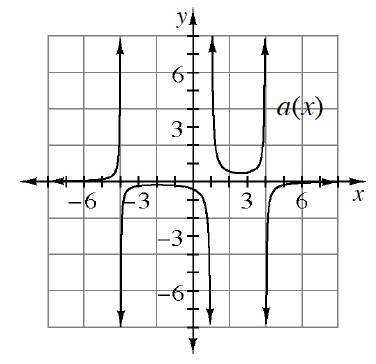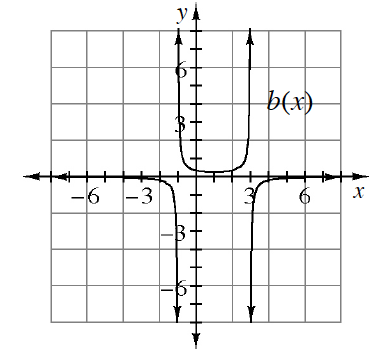### Home > PC > Chapter 5 > Lesson 5.1.3 > Problem5-39

5-39.

The graphs of $f\left(x\right)$, $g\left(x\right)$, and $h\left(x\right)$, are shown above. Below are the graphs of the reciprocal of two of the functions from above. Express $a(x)$ and $b(x)$ in terms of one of the functions listed above.

When $f(x)=0$, then $\frac{1}{f\left(x\right)}$ is undefined, creating a vertical asymptote.

1.1.1. Sketch the reciprocal of the function that was not used in parts (a) or (b).